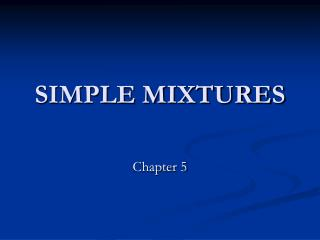DownloadDownload PresentationSIMPLE MIXTURES

# SIMPLE MIXTURES

Télécharger la présentation## SIMPLE MIXTURES

- - - - - - - - - - - - - - - - - - - - - - - - - - - E N D - - - - - - - - - - - - - - - - - - - - - - - - - - -
##### Presentation Transcript

1. SIMPLE MIXTURES Chapter 5

2. Simple Mixtures • Often in chemistry, we encounter mixtures of substances that can react together. • Chapter 7 deals with reactions, but let’s first deal with properties of mixtures that don’t react. • We shall mainly consider binary mixtures – mixtures of two components.

3. Partial Molar Volumes • Imagine a huge volume of pure water at 25 °C. If we add 1 mol H2O, the volume increases 18 cm3 (or 18 mL). • So, 18 cm3 mol-1 is the molar volume of pure water.

4. Partial Molar Volumes • Now imagine a huge volume of pure ethanol and add 1 mol of pure H2O it. How much does the total volume increase by?

5. Partial Molar Volumes • When 1 mol H2O is added to a large volume of pure ethanol, the total volume only increases by ~ 14 cm3. • The packing of water in pure water ethanol (i.e. the result of H-bonding interactions), results in only an increase of 14 cm3.

6. Partial Molar Volumes • The quantity 14 cm3 mol-1 is the partial molar volume of water in pure ethanol. • The partial molar volumes of the components of a mixture varies with composition as the molecular interactions varies as the composition changes from pure A to pure B.

7. Partial Molar Volumes • When a mixture is changed by dnA of A and dnB of B, then the total volume changes by:

8. Partial Molar Volumes

9. Partial Molar Volumes

10. Partial Molar Volumes

11. Partial Molar Volumes • How to measure partial molar volumes? • Measure dependence of the volume on composition. • Fit a function to data and determine the slope by differentiation.

12. Partial Molar Volumes • Ethanol is added to 1.000 kg of water. • The total volume, as measured by experiment, fits the following equation:

13. Partial Molar Volumes

14. Partial Molar Volumes • Molar volumes are always positive, but partial molar quantities need not be. The limiting partial molar volume of MgSO4 in water is -1.4 cm3mol-1, which means that the addition of 1 mol of MgSO4 to a large volume of water results in a decrease in volume of 1.4 cm3.

15. Partial Molar Gibbs energies The concept of partial molar quantities can be extended to any extensive state function. For a substance in a mixture, the chemical potential is defined as the partial molar Gibbs energy.

16. Partial Molar Gibbs energies For a pure substance:

17. Partial Molar Gibbs energies Using the same arguments for the derivation of partial molar volumes, Assumption: Constant pressure and temperature

18. Partial Molar Gibbs energies

19. Chemical Potential

20. Chemical Potential

21. Gibbs-Duhem equation

22. Gibbs-Duhem equation

23. Molarity and Molality Molarity, c, is the amount of solute divided by the volume of solution. Units of mol dm-3 or mol L-1. Molality, b, is the amount of solute divided by the mass of solvent. Units of mol kg-1.

24. Using Gibbs-Duhem The experimental values of partial molar volume of K2SO4(aq) at 298 K are found to fit the expression:

25. Using Gibbs-Duhem

26. Using Gibbs-Duhem

27. Using Gibbs-Duhem

28. Using Gibbs-Duhem

29. Using Gibbs-Duhem

30. Thermodynamics of mixing So we’ve seen how Gibbs energy of a mixture depends on composition. We know at constant temperature and pressure systems tend towards lower Gibbs energy. When we combine two ideal gases they mix spontaneously, so it must correspond to a decrease in G.

31. Thermodynamics of mixing

32. Dalton’s Law • The total pressure is the sum of all the partial pressure.

33. Thermodynamics of mixing

34. Thermodynamics of mixing

35. Thermodynamics of mixing

36. Thermodynamics of mixing

37. Gibbs energy of mixing A container is divided into two equal compartments. One contains 3.0 mol H2(g) at 25 °C; the other contains 1.0 mol N2(g) at 25 °C. Calculate the Gibbs energy of mixing when the partition is removed.

38. Gibbs energy of mixing Two processes: 1) Mixing 2) Changing pressures of the gases.

39. Other mixing functions

40. Other mixing functions

41. Ideal Solutions To discuss the equilibrium properties of liquid mixtures we need to know how the Gibbs energy of a liquid varies with composition. We use the fact that, at equilibrium, the chemical potential of a substance present as a vapor must be equal to its chemical potential in the liquid.

42. Ideal Solutions • If another substance is added to the pure liquid, the chemical potential of A will change. Chemical potential of vapor equals the chemical potential of the liquid at equilibrium.

43. Ideal Solutions